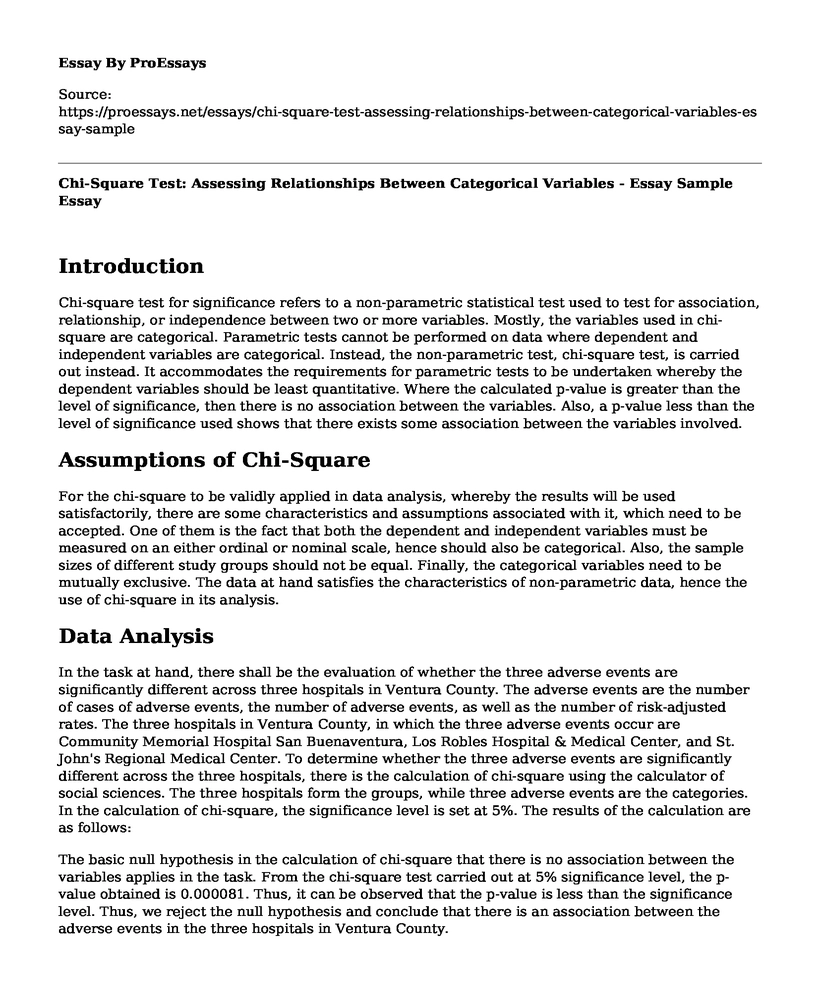# Chi-Square Test: Assessing Relationships Between Categorical Variables - Essay Sample

 Paper Type:  Essay Pages:  3 Wordcount:  552 Words Date:  2023-05-16
Categories:

## Introduction

Chi-square test for significance refers to a non-parametric statistical test used to test for association, relationship, or independence between two or more variables. Mostly, the variables used in chi-square are categorical. Parametric tests cannot be performed on data where dependent and independent variables are categorical. Instead, the non-parametric test, chi-square test, is carried out instead. It accommodates the requirements for parametric tests to be undertaken whereby the dependent variables should be least quantitative. Where the calculated p-value is greater than the level of significance, then there is no association between the variables. Also, a p-value less than the level of significance used shows that there exists some association between the variables involved.

Is your time best spent reading someone else’s essay? Get a 100% original essay FROM A CERTIFIED WRITER!

## Assumptions of Chi-Square

For the chi-square to be validly applied in data analysis, whereby the results will be used satisfactorily, there are some characteristics and assumptions associated with it, which need to be accepted. One of them is the fact that both the dependent and independent variables must be measured on an either ordinal or nominal scale, hence should also be categorical. Also, the sample sizes of different study groups should not be equal. Finally, the categorical variables need to be mutually exclusive. The data at hand satisfies the characteristics of non-parametric data, hence the use of chi-square in its analysis.

## Data Analysis

In the task at hand, there shall be the evaluation of whether the three adverse events are significantly different across three hospitals in Ventura County. The adverse events are the number of cases of adverse events, the number of adverse events, as well as the number of risk-adjusted rates. The three hospitals in Ventura County, in which the three adverse events occur are Community Memorial Hospital San Buenaventura, Los Robles Hospital & Medical Center, and St. John's Regional Medical Center. To determine whether the three adverse events are significantly different across the three hospitals, there is the calculation of chi-square using the calculator of social sciences. The three hospitals form the groups, while three adverse events are the categories. In the calculation of chi-square, the significance level is set at 5%. The results of the calculation are as follows:

The basic null hypothesis in the calculation of chi-square that there is no association between the variables applies in the task. From the chi-square test carried out at 5% significance level, the p-value obtained is 0.000081. Thus, it can be observed that the p-value is less than the significance level. Thus, we reject the null hypothesis and conclude that there is an association between the adverse events in the three hospitals in Ventura County.

## Conclusion

It can be established that non-parametric tests are essential in statistical tests. They help in the analysis and finally, interpretation of data that do not satisfy the assumptions laid for parametric tests. However, the power in which the parametric test is carried out is different from the power of predicting non-parametric tests. Therefore, parametric tests undertaken on all the data that satisfies all the assumptions have more power compared to non-parametric tests carried out to test similar results. However, the decision to either uses the parametric or non-parametric tests is entirely based on the features of data being analyzed for interpretation.

The analyzed data indicates that there exists some significant association between the three adverse events occurring in the three hospitals located in Ventura County.

Chi-Square Test: Assessing Relationships Between Categorical Variables - Essay Sample. (2023, May 16). Retrieved from https://proessays.net/essays/chi-square-test-assessing-relationships-between-categorical-variables-essay-sampleFree essays can be submitted by anyone,

so we do not vouch for their quality

Want a quality guarantee?
Order from one of our vetted writers instead

If you are the original author of this essay and no longer wish to have it published on the ProEssays website, please click below to request its removal:

Liked this essay sample but need an original one?

Hire a professional with VAST experience and 25% off!